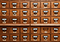# File and Directory Backup, Part 2

## Using Python and PyQt5Photo by Jan Antonin Kolar from Unsplash
`import sys, osfrom PyQt5.QtGui import QFont, QMoviefrom PyQt5.QtCore import Qt, QFileSystemWatcherfrom PyQt5.QtWidgets import QApplication, QLabel, QWidget`
`path = “C:/Users/mount/source/repos/MyDashboard/oo.txt”`
`class Spinner(QWidget):     def __init__(self):        super().__init__()        self.left   = 315        self.top    = 1        self.width  = 20        self.height = 23`
`def qblack(self):    self.setWindowFlags(Qt.FramelessWindowHint)    self.setGeometry(self.left,self.top,self.width,self.height)    self.setAutoFillBackground(True)    p = self.palette()    p.setColor(self.backgroundRole(), Qt.black)    self.setPalette(p)def qlabel(self):    return “QLabel {color: rgba(80,130,255,255); background-color: black;}”`
`qblack(self)center = Qt.AlignCenter canda_8 = QFont(‘Candalara’, 8)q_label = qlabel( self )`
`self.gif = QMovie(r’blu_circ.gif’)self.lbl = QLabel(self)self.lbl.setStyleSheet(q_label)self.lbl.setAlignment(center)self.lbl.setGeometry(0, 0, 25, 23)self.lbl.setMovie(self.gif)self.gif.start()`
`def write_txt_file(filename=’’, txt=’’, option=”a”):    with open(filename, option) as file:    file.write(‘\n’)    file.write(txt)`
`def xit(self):    write_txt_file(‘oo.txt’, ‘’, “w”)    self.close()`
`     count = 0     logg = log_directory()     lenn = len(dirs)     count += 1     if status == False:          ensure_directory( dst )         compare_directories( src, dst )         cmnd = f’robocopy {src} {dst} {swt}’         copi = subprocess.Popen( cmnd, shell=False )         code = copi.wait()      codes = range(0, 9)      if count == lenn and code in codes:         write_txt_file( ‘oo.txt’, ‘this file has changed!’ )  sys.exit()`
`codes = range(0, 9)`
`if code in codes:`
`if __name__ == ‘__main__’:    watcher = QFileSystemWatcher()    watcher.addPath(path)    app = QApplication([])    e = Spinner()    e.show()    watcher.fileChanged.connect(e.xit)    sys.exit(app.exec_())`
`import os os.system(r”start /min python backup.py”)os.system(r”start /min python backup_main.py”)`
`import sys, os, subprocessfrom qtpy_cfg import qblack, qlabel, write_text_filefrom PyQt5.QtGui import QFont, QMoviefrom PyQt5.QtCore import Qt, QFileSystemWatcherfrom PyQt5.QtWidgets import QApplication, QLabel, QWidget  path = “C:/Users/mount/source/repos/MyDashboard/oo.txt” class Spinner( QWidget ):‘’’  Creates a window for blu_circ.gif, a spinning indicator that shows the backup operation is being performed ‘’’     def __init__(self):        super().__init__()        self.left = 315        self.top = 1        self.width = 20        self.height = 23         qblack( self )      # PyQt5 window initialization function        center = Qt.AlignCenter        canda_8 = QFont(‘Candalara’, 8)        q_label = qlabel( self ) # PyQt5 label stylesheet function         self.gif = QMovie( r’blu_circ.gif’ )        self.lbl = QLabel( self )        self.lbl.setStyleSheet( q_label )        self.lbl.setAlignment( center )        self.lbl.setFont( canda_8 )        self.lbl.setGeometry( 0, 0, 25, 23 )        self.lbl.setMovie( self.gif )        self.gif.start()      def xit(self):    ‘’’     Resets the status-check file ‘oo.txt’    to a blank file and closes the spinner     '’’        write_txt_file(‘oo.txt’, ‘’, “w”)        self.close()   if __name__ == ‘__main__’: watcher = QFileSystemWatcher() watcher.addPath( path ) # Path to the watched file app = QApplication( [] ) e = Spinner() e.show() watcher.fileChanged.connect( e.xit ) sys.exit( app.exec_() )`
`import osimport sysimport timeimport filecmpimport subprocess   dirs = (     (r”C:\Users\mount\source\repos”, r”M:\_BACKUP\REPOS”),     (r”D:\mount\Downloads”, r”M:\_BACKUP\DOWNLOADS”),     (r”D:\mount\Documents”, r”M:\_BACKUP\DOCUMENTS”),     (r”D:\Internet-Marketing”, r”M:\_BACKUP\IM”),     (r”D:\_HOLOSYNC”, r”M:\_BACKUP\HOLOSYNC”),     (r”D:\mount\Music”, r”M:\_BACKUP\MUSIC”),     (r”D:\_BIZ”, r”M:\_BACKUP\BIZ”),     (r”D:\_PWA”, r”M:\_BACKUP\PWA”),     (r”C:\data\db”, r”M:\_BACKUP\DB”),     (r”C:\ProgramData\MongoDB”, r”M:\_BACKUP\MONGODB “), )   def ensure_directory( dst ): ‘’’  If the destination folder doesn’t exist, create it  ‘’’      directory = os.path.dirname( dst )     if not os.path.exists( directory ):         os.makedirs( directory )    def compare_directories( src, dst ): ‘’’  Compares the source and destination directories. Returns False if not the same. True means both directories and files match and no backup is required.  ‘’’      try:         comp = filecmp.dircmp( src, dst )         common = sorted( comp.common )     except:         return False      left = sorted( comp.left_list )     right = sorted( comp.right_list )     if left != common or right != common:         return False      if len( comp.diff_files ):         return False      for subdir in comp.common_dirs:     left_subdir = os.path.join( src, subdir )     right_subdir = os.path.join( dst, subdir )     return compare_directories( left_subdir, right_subdir )  return True   def log_directory(): ‘’’  Creates a LOG directory, if it does not exist then creates a sub-directory named for date and time for the backup log  ‘’’     now = time.strftime(“%Y-%m-%d___%H-%M”)     direct = os.path.dirname(“C:\\Users\\mount\\source\\repos\\MyDashboard\\LOG\\”)     directory = direct + ‘\\’ + now + ‘\\’     if not os.path.exists( directory ):         os.makedirs( directory ) return directory   count = 0 logg = log_directory() lenn = len(dirs)  for dir in dirs: ''' src = source directory dst = destination directory swt = switches for robocopy: /xo = only newer versions of file, /s = all occupied sub-directories, /MT:nn = # of threads (maximum=128, default=8) /xx = copy source file even when destination file does not exist /LOG+ = a log is created for every dir in dirs. /LOG+ appends all logs to one file /r:n = number of times to retry, default = 1,000,000 ### you WILL want to use this switch /w:nn = number of seconds to wait before retrying, default is 30 '''     count += 1     src, dst, swt = dir, dir, f”/XX /r:2 /xo /s /w:5 /MT:128 /LOG+:{logg}_BACKUP.log”     status = (compare_directories(src, dst))       if status == False:          ensure_directory( dst )         compare_directories( src, dst )         cmnd = f’robocopy {src} {dst} {swt}’         copi = subprocess.Popen( cmnd, shell=False )        code = copi.wait()      codes = range(0, 9)      if count == lenn and code in codes:         write_txt_file( ‘oo.txt’, ‘this file has changed!’ )  sys.exit()`

Retired, Self taught in python